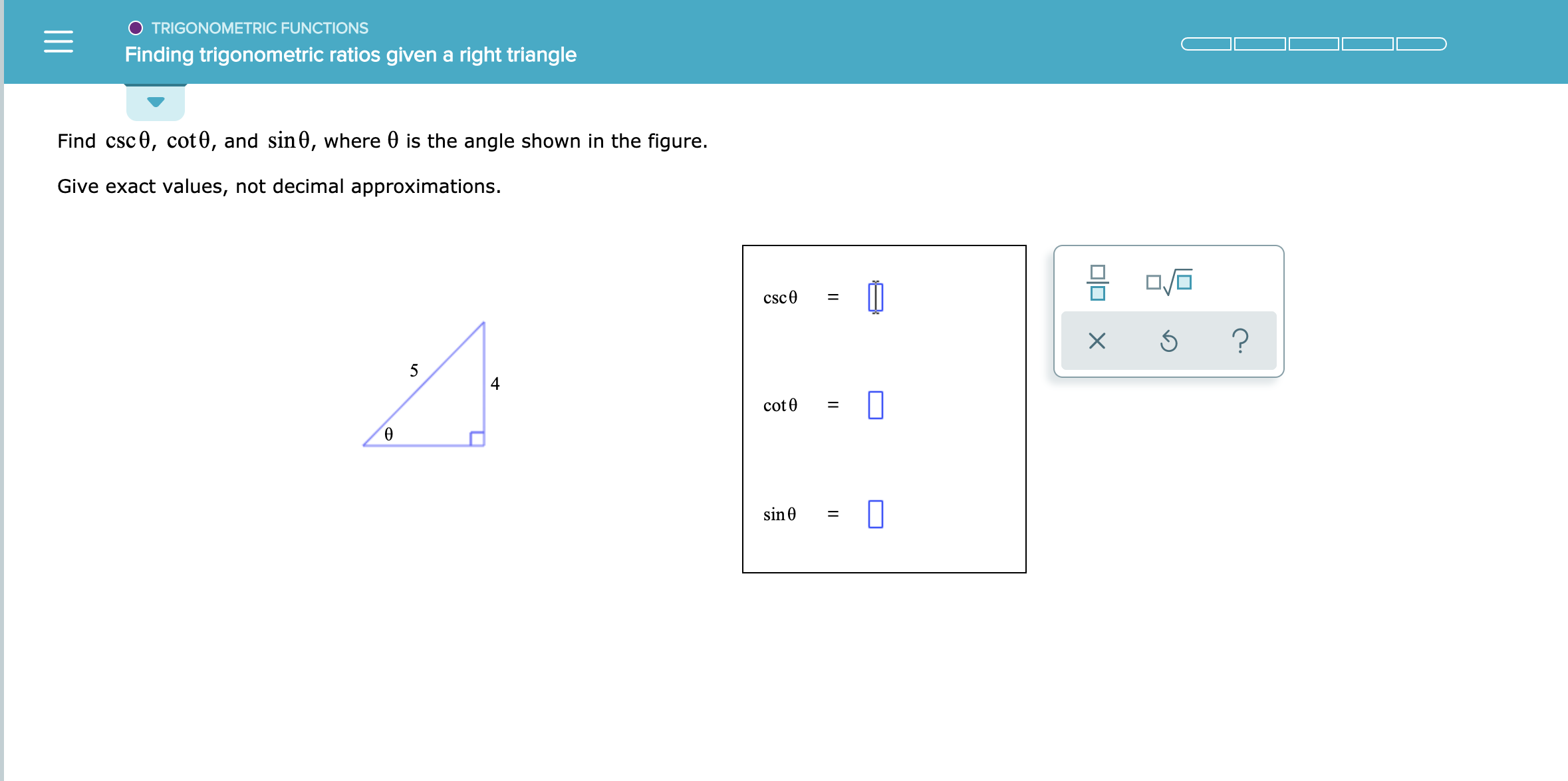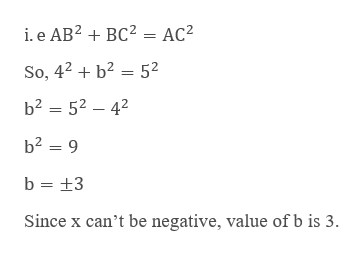# TRIGONOMETRIC FUNCTIONSFinding trigonometric ratios given a right triangleFind csc0, cot0, and sin0, where 0 is the angle shown in the figure.Give exact values, not decimal approximations.csc0?54cot0sin0

Question
1 views

See attachedhelp_outlineImage TranscriptioncloseTRIGONOMETRIC FUNCTIONS Finding trigonometric ratios given a right triangle Find csc0, cot0, and sin0, where 0 is the angle shown in the figure. Give exact values, not decimal approximations. csc0 ? 5 4 cot0 sin0 fullscreen
check_circle

Step 1

Let the given triangle be ABC with sides BC = a = 4 and AC = c = 5.

Step 2

To Find:

Step 3

First, we find the missing side BC by...help_outlineImage Transcriptionclosei. e AB2BC2 = AC2 So, 42b2 52 b2 52 42 b2 9 b +3 Since x can't be negative, value of b is 3. fullscreen

### Want to see the full answer?

See Solution

#### Want to see this answer and more?

Solutions are written by subject experts who are available 24/7. Questions are typically answered within 1 hour.*

See Solution
*Response times may vary by subject and question.
Tagged in

### Other